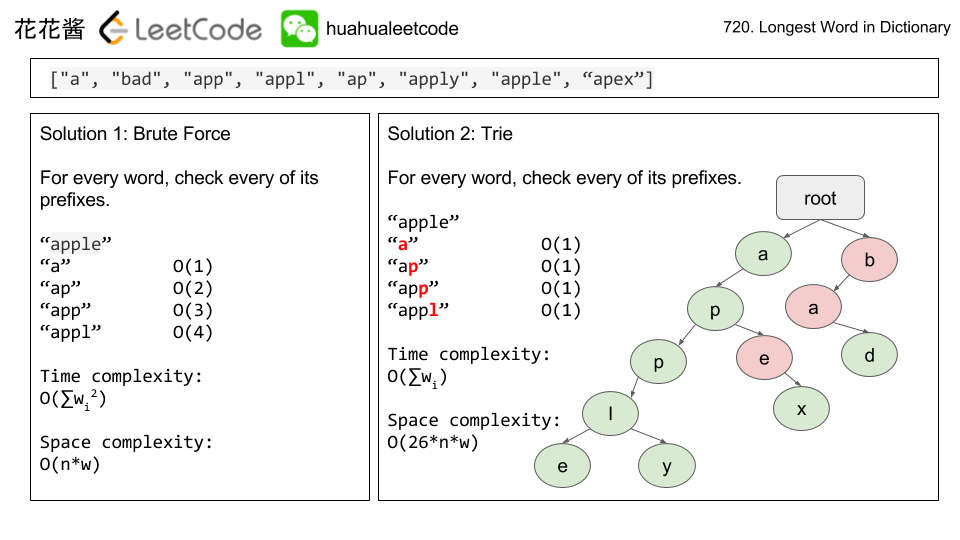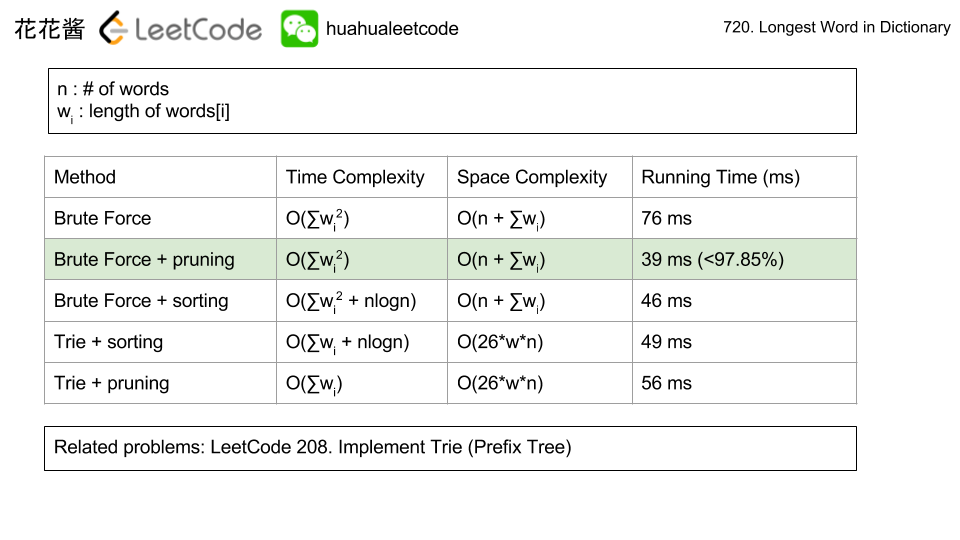# Posts tagged as “trie”

Given a 2D board and a list of words from the dictionary, find all words in the board.

Each word must be constructed from letters of sequentially adjacent cell, where “adjacent” cells are those horizontally or vertically neighboring. The same letter cell may not be used more than once in a word.

Example:

Input:
board = [
['o','a','a','n'],
['e','t','a','e'],
['i','h','k','r'],
['i','f','l','v']
]
words = ["oath","pea","eat","rain"]

Output: ["eat","oath"]


Note:

1. All inputs are consist of lowercase letters a-z.
2. The values of words are distinct.

## Solution 1: DFS

Time complexity: O(sum(m*n*4^l))
Space complexity: O(l)

## Solution 2: Trie

Store all the words into a trie, search the board using DFS, paths must be in the trie otherwise there is no need to explore.

Time complexity: O(sum(l) + 4^max(l))
space complexity: O(sum(l) + l)

## LeetCode 1029 Two City Scheduling

Solution1: DP

dp[i][j] := min cost to put j people into city A for the first i people
dp = 0
dp[i] = dp[i -1] + cost_b
dp[i][j] = min(dp[i – 1][j] + cost_b, dp[i – 1][j – 1] + cost_a)
ans := dp[n][n/2]

Time complexity: O(n^2)
Space complexity: O(n^2)

## C++

Solution 2: Greedy

Sort by cost_a – cost_b

Choose the first n/2 people for A, rest for B

Time complexity: O(nlogn)
Space complexity: O(1)

## 1030. Matrix Cells in Distance Order

Solution: Sorting

Time complexity: O(RC*log(RC))
Space complexity: O(RC)

## 1031. Maximum Sum of Two Non-Overlapping Subarrays

Solution: Prefix sum

Time complexity: O(n^2)
Space complexity: O(n)

## 1032. Stream of Characters

Solution: Trie

Time complexity:

• build O(sum(len(w))
• query O(max(len(w))

Space complexity: O(sum(len(w))

# Problem

https://leetcode.com/problems/replace-words/description/

In English, we have a concept called root, which can be followed by some other words to form another longer word – let’s call this word successor. For example, the root an, followed by other, which can form another word another.

Now, given a dictionary consisting of many roots and a sentence. You need to replace all the successor in the sentence with the root forming it. If a successor has many roots can form it, replace it with the root with the shortest length.

You need to output the sentence after the replacement.

Example 1:

Input: dict = ["cat", "bat", "rat"]
sentence = "the cattle was rattled by the battery"
Output: "the cat was rat by the bat"


Note:

1. The input will only have lower-case letters.
2. 1 <= dict words number <= 1000
3. 1 <= sentence words number <= 1000
4. 1 <= root length <= 100
5. 1 <= sentence words length <= 1000

# Solution 1: HashTable

Time complexity: O(sum(w^2))

Space complexity: O(sum(l))

## Solution2: Trie

Time complexity: O(sum(l) + n)

Space complexity: O(sum(l) * 26)

Problem:

Given many wordswords[i] has weight i.

Design a class WordFilter that supports one function, WordFilter.f(String prefix, String suffix). It will return the word with given prefix and suffix with maximum weight. If no word exists, return -1.

Examples:

Note:

1. words has length in range [1, 15000].
2. For each test case, up to words.length queries WordFilter.f may be made.
3. words[i] has length in range [1, 10].
4. prefix, suffix have lengths in range [0, 10].
5. words[i] and prefix, suffix queries consist of lowercase letters only.

Idea:

Construct all possible filters

Solution1:

C++

Time complexity: O(NL^3 + QL)  where N is the number of words, L is the max length of the word, Q is the number of queries.

Space complexity: O(NL^3)

Version #2

Solution 2:

C++ / Trie

Time complexity: O(NL^2 + QL)  where N is the number of words, L is the max length of the word, Q is the number of queries.

Space complexity: O(NL^2)

Related Problems:

Given a list of strings words representing an English Dictionary, find the longest word in words that can be built one character at a time by other words in words. If there is more than one possible answer, return the longest word with the smallest lexicographical order.

If there is no answer, return the empty string.

Example 1:

Example 2:

Note:

• All the strings in the input will only contain lowercase letters.
• The length of words will be in the range [1, 1000].
• The length of words[i] will be in the range [1, 30].

Idea:

Brute force

TrieSolution:

C++

Trie + Sorting

Trie + No sorting

Mission News Theme by Compete Themes.